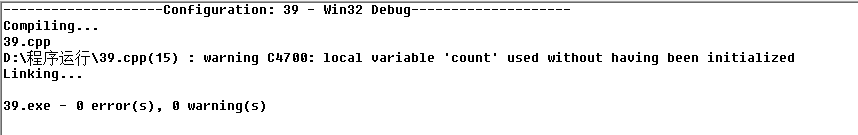•闪存
•博客
• 发言小组
• 投递新闻
• 提问博问
• 添加收藏
•文库

# 使用函数输出指定范围内的完数

0
[已解决问题] 解决于 2018-08-08 16:53#include<stdio.h>
int factorsum(int i);
int main(){
int n,m,i,count;
printf("Enter m,n:");
scanf("%d%d",&m,&n);

if(m>n){

}
printf("Enter m,n:");
scanf("%d%d",&m,&n);
if(m<n){
for(i=m;i<=n;i++){
factorsum(i);
if(count==i){
printf("%d\n",i);
}
}
}
return 0;
}
int factorsum(int i){
int j,count;
count=0;
for(j=1;j<=i/2;j++){
if(i%j==0){
count=count+j;
}
}
return count;
}

Enter m,n:6 3
Enter m,n:3 6
3
4
5
6
Press any key to continue

0

if(count==i)@西戈wzc:

count = factorsum(i);

@窗户: 就是就是，我也发现这个问题了，子函数只是运行却没有返回值

0

您需要登录以后才能回答，未注册用户请先注册